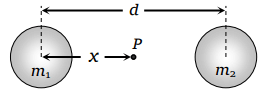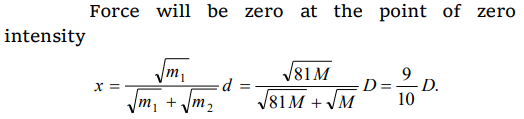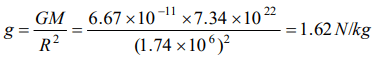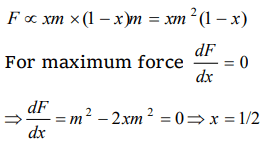## Gravitation Questions and Answers Part-2

1. The gravitational force between two point masses $m_{1}$ and $m_{2}$ at separation r is given by$F=k\frac{m_{1}m_{2}}{r^{2}}$   . The constant k
a) Depends on system of units only
b) Depends on medium between masses only
c) Depends on both (a) and (b)
d) Is independent of both (a) and (b)

Explanation: k represents gravitational constant which depends only on the system of units

2. The distance of the centres of moon and earth is D. The mass of earth is 81 times the mass of the moon. At what distance from the centre of the earth, the gravitational force will be zero
a) $\frac{D}{2}$
b) $\frac{2D}{3}$
c) $\frac{4D}{3}$
d) $\frac{9D}{10}$

Explanation:3.Who among the following gave first the experimental value of G
a) Cavendish
b) Copernicus
c) Brook Teylor
d) None of these

Explanation: Cavendish

4. The mass of the moon is $7.34\times10^{22}kg$   and the radius is $1.74\times10^{6}m$   . The value of gravitation force will be
a) 1.45 N/kg
b) 1.55 N/kg
c) 1.75 N/kg
d) 1.62 N/kg

Explanation:5.The centripetal force acting on a satellite orbiting round the earth and the gravitational force of earth acting on the satellite both equal F. The net force on the satellite is
a) Zero
b) F
c) $F\sqrt{2}$
d) 2 F

Explanation: Actually gravitational force provides the centripetal force

6. Reason of weightlessness in a satellite is
a) Zero gravity
b) Centre of mass
c) Zero reaction force by satellite surface
d) None

Explanation: Zero reaction force by satellite surface

7. Mass M is divided into two parts xM and (1 - x)M . For a given separation, the value of x for which the gravitational attraction between the two pieces becomes maximum is
a) $\frac{1}{2}$
b) $\frac{3}{5}$
c) 1
d) 2

Explanation:8. The force of gravitation is
a) Repulsive
b) Electrostatic
c) Conservative
d) Non-conservative

Explanation: The force of gravitation is Conservative

9.The gravitational force $F_{g}$ between two objects does not depend on
a) Sum of the masses
b) Product of the masses
c) Gravitational constant
d) Distance between the masses

b) $\frac{F}{3}$
c) $\frac{F}{9}$